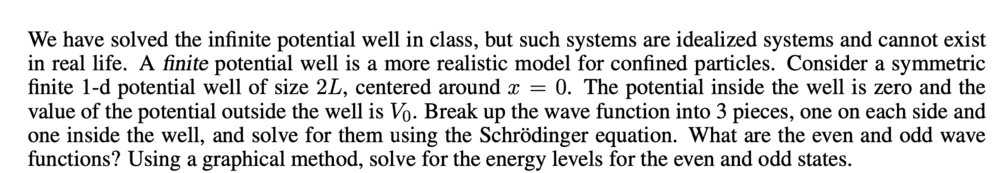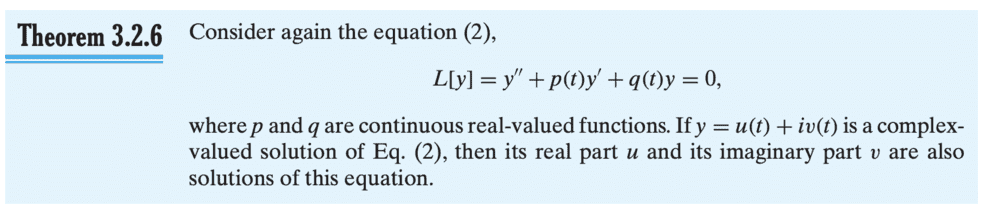# Finite potential well

docnet
Homework Statement:
Relevant Equations:To find the energy states of the particle, we define the wave function over three discrete domains defined by the sets ##\left\{x<-L\right\}##, ##\left\{L<x\right\}## and ##\left\{|x|<L\right\}##. The time independent Schr\"odinder equation is
\begin{equation}\frac{\hbar^2}{2m}\frac{d^2\psi}{dx^2}=[E-V(x)]\psi
\end{equation}
Outside of the finite well, the ##V(x)## term vanishes to zero to give the following linear second order ODE
\begin{equation}\frac{d^2\psi}{dx^2}=\frac{2m}{\hbar^2}|E|\psi
\end{equation}
whose characteristic polynomial is
\begin{equation}r^2-\frac{2m|E|}{\hbar^2}=0\end{equation} with the corresponding roots \begin{equation}r=\pm \sqrt{\frac{2m|E|}{\hbar^2}}=\pm \alpha\end{equation}
The general solution to the ODE is
\begin{equation}
\psi=C_1e^{\alpha x}+C_2e^{-\alpha x}
\end{equation}
To obtain the wave function inside of the finite well, we set ##V(x)=V_0## and solve the following time independent Schr\"odinder equation
\begin{equation}
\frac{d^2\psi}{dx^2}=\frac{2m}{\hbar^2}[V_0-|E|]\psi
\end{equation}
whose characteristic polynomial is
\begin{equation}
r^2+\frac{2m}{\hbar^2}[V_0-|E|]=0
\end{equation}
with the corresponding roots
\begin{equation}
r=\pm \sqrt{\frac{-2m}{\hbar^2}[V_0-|E|]}\Rightarrow\pm i\sqrt{q}
\end{equation}
The general solution to the ODE is
\begin{equation}
\psi=C_1e^{iqx}+C_2e^{-iqx}\Rightarrow \psi= C_3cos(qx)+iC_4sin(qx)
\end{equation}
Taking only the real parts of ##\psi## we have
\begin{equation}
\psi=A_1cos(qx)+A_2sin(qx)
\end{equation}
So the even parity wave functions are

\begin{equation}
\psi=Ce^{\alpha x}|_{\left\{x<-L\right\}}
\end{equation}
\begin{equation}
\psi=Acos(qx)|_{\left\{|x|<L\right\}}
\end{equation}
\begin{equation}
\psi=Ce^{-\alpha x}|_{\left\{L<x\right\}}
\end{equation}
and the odd parity wave functions are
\begin{equation}
\psi=-Ce^{\alpha x}|_{\left\{x<-L\right\}}
\end{equation}
\begin{equation}
\psi=Asin(qx)|_{\left\{|x|<L\right\}}
\end{equation}
\begin{equation}
\psi=Ce^{-\alpha x}|_{\left\{L<x\right\}}
\end{equation}
For even solutions, we impose the boundary condition ##\psi \in C^1(\pm L)## such that ##\psi## is continuous and once differentiable at ##x=\pm L##
\begin{equation}
\psi_{in}(\pm L)= \psi_{out}(\pm L)\Rightarrow Acos(qL)=Ce^{-\alpha L}
\end{equation}
\begin{equation}
\psi'_{in}(\pm L)= \psi'_{out}(\pm L)\Rightarrow Aqsin(qL)=\alpha Ce^{-\alpha L}
\end{equation}
We divide equation (16) by (15) and then make substitutions using $$y=qL$$ and $$r=\sqrt{\frac{2mV_0L^2}{\hbar^2}}$$ The solutions to the energy states are given implicitly by the following expression.
\begin{equation}
ytan(y)=\sqrt{r^2-y^2}
\end{equation}
We impose the same boundary condition on the odd functions
\begin{equation}
\psi_{in}(\pm L)= \psi_{out}(\pm L)\Rightarrow Asin(qL)=Ce^{-\alpha L}
\end{equation}
\begin{equation}
\psi'_{in}(\pm L)= \psi'_{out}(\pm L)\Rightarrow Aqcos(qL)=-\alpha Ce^{-\alpha L}
\end{equation}
We divide equation (20) by (21), make the same substitutions as above, and obtain the following expression.
\begin{equation}
-ycot(y)=\sqrt{r^2-y^2}
\end{equation}

The expressions do not have analytical solutions, so there are no closed forms of the energy levels of the even and odd states. However, given values for ##V_o## and ##L##, we can solve by graphing the curves given implicitly by the formulas.

Staff Emeritus
Homework Helper
It looks like you got the potential backwards. It's zero inside the well and ##V_0 > 0## outside the well.

•docnet
docnet
To find the energy states of the particle, we define the wave function over three discrete domains defined by the sets ##\left\{x<-L\right\}##, ##\left\{L<x\right\}## and ##\left\{|x|<L\right\}##. The time independent Schrodinder equation is
\begin{equation}\frac{-\hbar^2}{2m}\frac{d^2\psi}{dx^2}=[E-V(x)]\psi
\end{equation}
Outside of the finite well, ##V(x)=V_0## gives the following linear second order ODE
\begin{equation}\frac{d^2\psi}{dx^2}=\frac{2m}{\hbar^2}[V_0-|E|]\psi
\end{equation}
whose characteristic polynomial is
\begin{equation}r^2-\frac{2m[V_0-|E|]}{\hbar^2}=0\end{equation} with the corresponding roots \begin{equation}r=\pm \sqrt{\frac{2m[V_0-|E|]}{\hbar^2}}=\pm \alpha\end{equation}
The general solution to the ODE is
\begin{equation}
\psi=C_1e^{\alpha x}+C_2e^{-\alpha x}
\end{equation}
To obtain the wave function inside of the finite well, we set ##V(x)=0## and solve the following time independent Schrodinder equation
\begin{equation}
\frac{d^2\psi}{dx^2}=\frac{-2m}{\hbar^2}|E|\psi
\end{equation}
whose characteristic polynomial is
\begin{equation}
r^2+\frac{2m}{\hbar^2}|E|=0
\end{equation}
with the corresponding roots
\begin{equation}
r=\pm \sqrt{\frac{-2m}{\hbar^2}|E|}\Rightarrow\pm i\sqrt{q}
\end{equation}
The general solution to the ODE is
\begin{equation}
\psi=C_1e^{iqx}+C_2e^{-iqx}\Rightarrow \psi= C_3cos(qx)+iC_4sin(qx)
\end{equation}
The real parts of ##\psi## are a solution to our ODE
\begin{equation}
\psi=A_1cos(qx)+A_2sin(qx)
\end{equation}
So the even parity wave functions are

\begin{equation}
\psi=Ce^{\alpha x}|_{\left\{x<-L\right\}}
\end{equation}
\begin{equation}
\psi=Acos(qx)|_{\left\{|x|<L\right\}}
\end{equation}
\begin{equation}
\psi=Ce^{-\alpha x}|_{\left\{L<x\right\}}
\end{equation}
and the odd parity wave functions are
\begin{equation}
\psi=-Ce^{\alpha x}|_{\left\{x<-L\right\}}
\end{equation}
\begin{equation}
\psi=Asin(qx)|_{\left\{|x|<L\right\}}
\end{equation}
\begin{equation}
\psi=Ce^{-\alpha x}|_{\left\{L<x\right\}}
\end{equation}
For even solutions, we impose the boundary condition ##\psi \in C^1(\pm L)## such that ##\psi## is continuous and once differentiable at ##x=\pm L##
\begin{equation}
\psi_{in}(\pm L)= \psi_{out}(\pm L)\Rightarrow Acos(qL)=Ce^{-\alpha L}
\end{equation}
\begin{equation}
\psi'_{in}(\pm L)= \psi'_{out}(\pm L)\Rightarrow Aqsin(qL)=\alpha Ce^{-\alpha L}
\end{equation}
We divide equation (16) by (15) and then make substitutions using $$y=qL$$ and $$r=\sqrt{\frac{2mV_0L^2}{\hbar^2}}$$ The solutions to the even functions are given implicitly by the following expression.
\begin{equation}
ytan(y)=\sqrt{r^2-y^2}
\end{equation}
We impose the same boundary condition on the odd functions
\begin{equation}
\psi_{in}(\pm L)= \psi_{out}(\pm L)\Rightarrow Asin(qL)=Ce^{-\alpha L}
\end{equation}
\begin{equation}
\psi'_{in}(\pm L)= \psi'_{out}(\pm L)\Rightarrow Aqcos(qL)=-\alpha Ce^{-\alpha L}
\end{equation}
and divide equation (20) by (21). Then, we make the same substitutions as above to obtain the following expression.
\begin{equation}
-ycot(y)=\sqrt{r^2-y^2}
\end{equation}
The expressions do not have analytical solutions, so there are no closed forms of the energy levels of the even and odd states.

Staff Emeritus
Homework Helper
When you solve for the wave function inside the well, it's not so much that you take only the real part of the solution but you choose the arbitrary constants so that the wave function is real.

•docnet
docnet
When you solve for the wave function inside the well, it's not so much that you take only the real part of the solution but you choose the arbitrary constants so that the wave function is real.
Thanks. It turns out I misquoted a theorem I read many months ago. I was supposed to write the real and imaginary parts of the complex valued solution form a fundamental set of solutions to the ODE. I went back to my ODE textbook and found the following theorem that I was trying to remember.docnet
Question: since the Schrodinger equation has a second derivative operator, shouldn't the boundary condition for the wavefunction be that ##\psi## is twice continuously differentiable at the boundary, not just once continuously differentiable?

Last edited:
Staff Emeritus
Homework Helper
Classically, if you have a step potential, the momentum of the particle changes abruptly at the boundary. Physically, an infinite force imparts a finite momentum to the particle, and the kinetic energy of the particle is undefined at the boundary. In quantum mechanics, it's similar, so the second derivative of the wave function isn't defined at the boundary.

Note you have a similar situation with the infinite square well. In that problem, you don't even require the first derivative to be continuous at the boundaries because of the infinite potential outside the well. With the finite square well, you've gotten rid of that infinity so you can again require ##\psi'## be continuous, but there's still the remaining infinity in ##V'(x=\pm L)##.

••docnet and PeroK
docnet
Classically, if you have a step potential, the momentum of the particle changes abruptly at the boundary. Physically, an infinite force imparts a finite momentum to the particle, and the kinetic energy of the particle is undefined at the boundary. In quantum mechanics, it's similar, so the second derivative of the wave function isn't defined at the boundary.

Note you have a similar situation with the infinite square well. In that problem, you don't even require the first derivative to be continuous at the boundaries because of the infinite potential outside the well. With the finite square well, you've gotten rid of that infinity so you can again require ##\psi'## be continuous, but there's still the remaining infinity in ##V'(x=\pm L)##.
wow! thanks for the explanation.